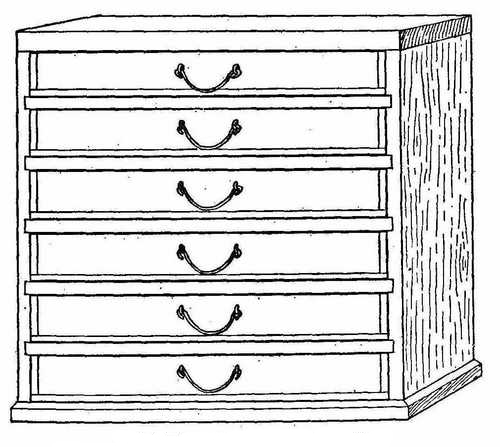# Data Types

 What does Data Type mean? Every Programming language have different names for the memory representation of data. For example, if the memory holds numbers between -2147483648 to 2147483647, most programming languages name this data as the "Integer" data type. Variables? Variables are names that refer to the sections of memory that store data. To help understand this concept, imagine the memory as a cabinet (Figure 1) with drawers of different size. The drawer size is the memory storage area required in bytes..
Figure 1 - Cabinet

• In order to use a drawer to store data, you first have to tell the computer that you want to use the drawer; this process is known as a declaration.
• In the declaration phase, you use a specific word to tell the computer what kind and size of drawer you want to use; this word is known as a keyword.
• And just as for any newborn baby, you have to give the drawer a name (it helps if you give it a meaningful name); this name is known as the variable constant.
• Data is placed into a drawer by assigning the data to the drawer.
• You can set the value of the created variable (drawer) in the same declaration line; this process is known as initialization.

When we create a variable, we tell the computer that we want it to assign a specified memory length (in bytes) to the variable. This is because a simple number, a letter or a large number will all occupy different amounts of space when stored in memory, so the computer needs to ask us to identify the kind of data and its length. This is what the data type is for.

For example, if we talk to the computer using this line of code: